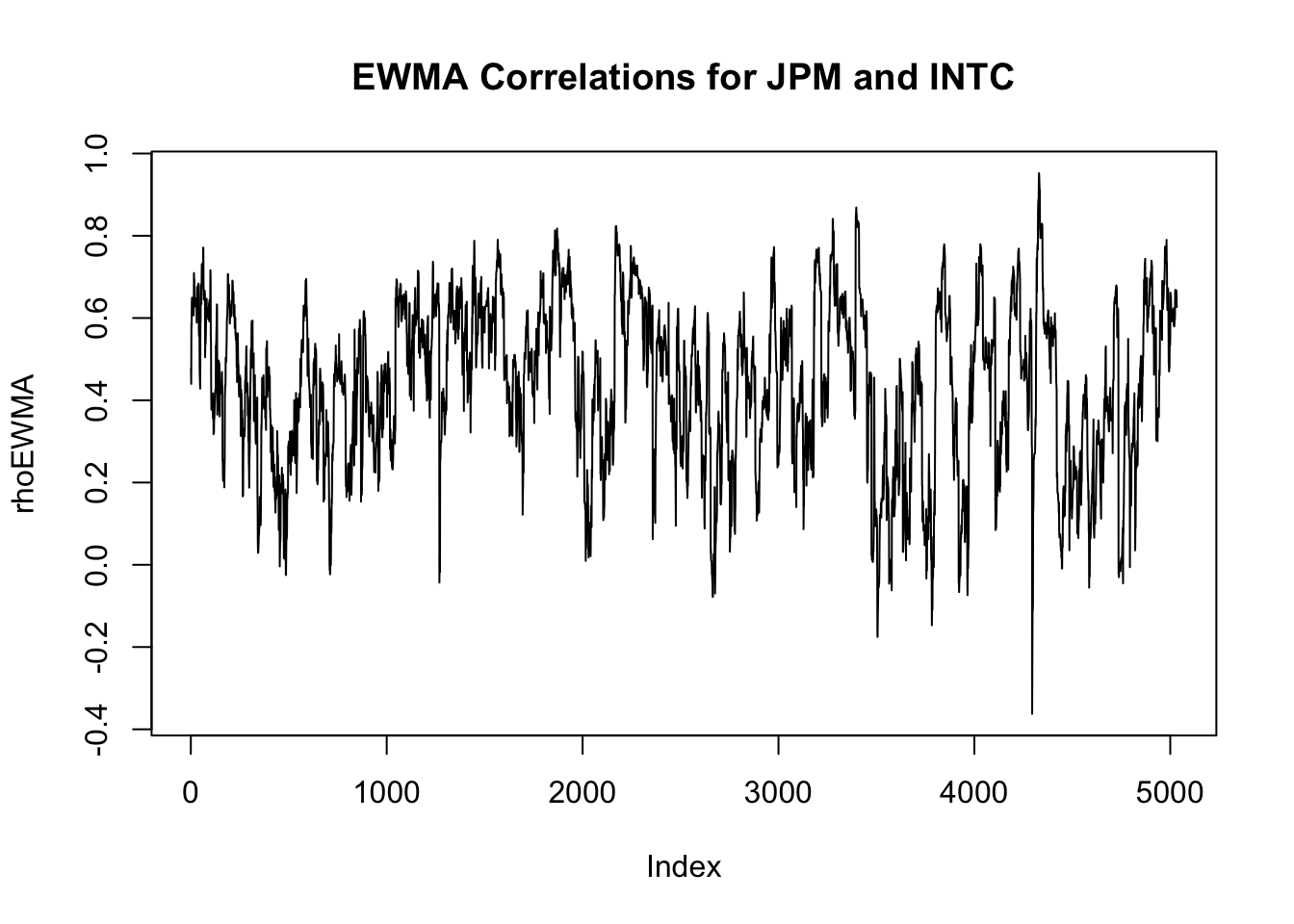# 13Multivariate volatility

Below we address implementation of volatility models as discussed in chapter three of the book chapter3

GARCH models have to be solved using maximum likelihood methods, how the GARCH packages, like `rmgarch`, fit their models, discussed in Chapter 11. For the mathematical details, see the book or slides, see package documentation for how to use `rmgarch`.

We use maximum likelihood methods for estimating volatility models, and use the R package `rmgarch` for the actual implementation. See the package documentation for more details, manual and a more detailed vignette. It is developed by Alexios Galanos and is constantly maintained and updated. The development code can be found on github.

## 13.1 Libraries

``suppressPackageStartupMessages(library(rmgarch))``

## 13.2 Data

The data we use is `stocks.csv`.

``source('common/common.r')``
``````load('data/data.RData')
Return=data\$Return
Ticker=data\$Ticker``````

## 13.3 EWMA

``````y=as.matrix(Return[,c("JPM","INTC")])
EWMA = matrix(nrow=dim(y),ncol=3)
lambda = 0.94
S = cov(y)
EWMA[1,] = c(S)[c(1,4,2)]
for (i in 2:dim(y)){
S = lambda*S+(1-lambda)*
y[i-1,] %*% t(y[i-1,])
EWMA[i,] = c(S)[c(1,4,2)]
}
rhoEWMA = EWMA[,3]/sqrt(EWMA[,1]*EWMA[,2])
plot(rhoEWMA,type='l',main="EWMA Correlations for JPM and INTC")``````## 13.4 DCC

### 13.4.1`rmgarch`

The package `rmgarch` allows us easily estimate models with multiple assets in the same fashion as `rugarch`. The procedure is analogous, we first need to specify the model we want to use, and then fit it to the data. However, specifying the model has extra steps. To explain this, we will focus on the estimation of a DCC model.

In a DCC model, we assume that each individual asset follows some type of univariate model, usually a GARCH. And then we model the correlation between the assets using an ARMA-like process.

The process for fitting a DCC model using `rmgarch` is then:

1. Specify the univariate volatility model each asset follows using `ugarchspec()`;
2. Use the `multispec()` function to create a multivariate specification. This is essentially a list of univariate specifications. If we are going to use the same for every asset, we can use `replicate()`;
3. Then we need to create a `dccspec()` object, which takes in the list of univariate specifications for every asset, and the additional DCC joint specifications, like `dccOrder` and `distribution`;
4. Fit the specification to the data.

We will use the returns for JPM and Intel:

``y=as.matrix(Return[,c("JPM","INTC")])``

We will assume a simple GARCH(1,1) with mean zero for each stock. We will create a single univariate specification, and then replicate it into `multispec()`:

``````# Create the univariate specification
uni_spec = ugarchspec(
variance.model = list(
garchOrder = c(1,1)),
mean.model = list(
armaOrder = c(0,0),
include.mean = FALSE)
)
# Replicate it into a multispec element
mspec = multispec(replicate(2, uni_spec))``````

Let’s take a look inside the `mspec` object. We will see there are two univariate specifications, one per asset, and they are equal:

``mspec``
``````
*-----------------------------*
*     GARCH Multi-Spec        *
*-----------------------------*
Multiple Specifications : 2
Multi-Spec Type         : equal``````

You can check the specifications with `mspec@spec`

Now we proceed to create the specification for the DCC model using `dccspec()`:

``````spec = dccspec(
# Univariate specifications - Needs to be multispec
uspec = mspec,

# DCC specification. We will assume an ARMA(1,1)-like process
dccOrder = c(1,1),

# Distribution, here multivariate normal
distribution = "mvnorm"
)``````

We can call `spec` to see what is inside:

``spec``
``````
*------------------------------*
*       DCC GARCH Spec         *
*------------------------------*
Model          :  DCC(1,1)
Estimation     :  2-step
Distribution   :  mvnorm
No. Parameters :  9
No. Series     :  2``````

We can again see more details in `spec@model` and `spec@umodel`.

Now we can proceed to fit the specification to the data:

``````res = dccfit(spec, data = y)
res``````
``````
*---------------------------------*
*          DCC GARCH Fit          *
*---------------------------------*

Distribution         :  mvnorm
Model                :  DCC(1,1)
No. Parameters       :  9
[VAR GARCH DCC UncQ] : [0+6+2+1]
No. Series           :  2
No. Obs.             :  5034
Log-Likelihood       :  27227.76
Av.Log-Likelihood    :  5.41

Optimal Parameters
-----------------------------------
Estimate  Std. Error  t value Pr(>|t|)
[JPM].omega    0.000004    0.000003   1.5613  0.11846
[JPM].alpha1   0.092326    0.017139   5.3868  0.00000
[JPM].beta1    0.896338    0.020491  43.7438  0.00000
[INTC].omega   0.000004    0.000003   1.3599  0.17385
[INTC].alpha1  0.030623    0.004388   6.9782  0.00000
[INTC].beta1   0.959936    0.001737 552.5567  0.00000
[Joint]dcca1   0.009528    0.005876   1.6215  0.10491
[Joint]dccb1   0.973320    0.020506  47.4651  0.00000

Information Criteria
---------------------

Akaike       -10.814
Bayes        -10.802
Shibata      -10.814
Hannan-Quinn -10.810

Elapsed time : 1.188162 ``````

We can check what slots are inside:

``names(res@model)``
``````  "modelinc"      "modeldesc"     "modeldata"     "varmodel"
 "pars"          "start.pars"    "fixed.pars"    "maxgarchOrder"
 "maxdccOrder"   "pos.matrix"    "pidx"          "DCC"
 "mu"            "residuals"     "sigma"         "mpars"
 "ipars"         "midx"          "eidx"          "umodel"       ``````
``names(res@mfit)``
``````  "coef"            "matcoef"         "garchnames"      "dccnames"
 "cvar"            "scores"          "R"               "H"
 "Q"               "stdresid"        "llh"             "log.likelihoods"
 "timer"           "convergence"     "Nbar"            "Qbar"
 "plik"           ``````
``````# Coefficient matrix
res@mfit\$matcoef``````
``````                  Estimate   Std. Error    t value     Pr(>|t|)
[JPM].omega   4.320478e-06 2.767254e-06   1.561287 1.184561e-01
[JPM].alpha1  9.232608e-02 1.713926e-02   5.386819 7.171575e-08
[JPM].beta1   8.963376e-01 2.049062e-02  43.743801 0.000000e+00
[INTC].omega  3.501196e-06 2.574546e-06   1.359927 1.738529e-01
[INTC].alpha1 3.062290e-02 4.388355e-03   6.978219 2.989387e-12
[INTC].beta1  9.599362e-01 1.737263e-03 552.556727 0.000000e+00
[Joint]dcca1  9.528089e-03 5.876088e-03   1.621502 1.049100e-01
[Joint]dccb1  9.733201e-01 2.050600e-02  47.465136 0.000000e+00``````
``````# Log likelihood
res@mfit\$llh``````
`` 27227.76``

The matrix H inside `res@mfit` includes the covariances. It is 3-dimensional, since it includes the 2x2 covariance matrix for each of the T time periods:

``````H = res@mfit\$H
dim(H)``````
``    2    2 5034``
``````# First period's covariances
H[,,1]``````
``````             [,1]         [,2]
[1,] 0.0005220625 0.0001946885
[2,] 0.0001946885 0.0003907303``````

We can extract the conditional correlation in two ways. One is computing it from H:

``````# Initializing the vector
rhoDCC = vector(length = dim(y))

# Populate with the correlations
rhoDCC = H[1,2,] / sqrt(H[1,1,]*H[2,2,])``````

## 13.5 Compare EWMA and DCC

``````par(mar=c(2,4,2,0))
matplot(cbind(rhoEWMA,rhoDCC),
type='l',
bty='l',
lty=1,
col=c("green","blue"),
main="EWMA and DCC correlations for JPM and C",
ylab="Correlations",
las=1
)
legend("bottomright",
legend=c("EWMA","DCC"),
lty=1,
col=c("green","blue"),
bty='n'
)``````## 13.6 Exercise

Following the steps specified in the slides, construct the conditional variance matrix for JPM and INTEL by using the constant conditional correlations (CCC) model. Compute the constant conditional correlation.

``````# Answer
library(rugarch)
uni_spec = ugarchspec(
variance.model = list(
garchOrder = c(1,1)),
mean.model = list(
armaOrder = c(0,0),
include.mean = FALSE)
)
garch.fit1=ugarchfit(spec=uni_spec, data=y[,1])
garch.fit2=ugarchfit(spec=uni_spec, data=y[,2])
sigma1=as.numeric(sigma(garch.fit1))
sigma2=as.numeric(sigma(garch.fit2))

epsilon=c()
for (i in 1:length(sigma1)){
epsilon=cbind(epsilon,solve(diag(c(sigma1[i],sigma2[i])))%*%y[i,])
}
r.mat=epsilon%*%t(epsilon)/length(sigma1)
cvm=array(NA, dim=c(2,2,length(sigma1)))  # conditional variance matrix (array)
for (i in 1:length(sigma1)){
dt=diag(c(sigma1[i],sigma2[i]))
cvm[,,i]=dt%*%r.mat%*%dt
}
cvm[,,1] # conditional variance matrix at t=1
rhoCCC=cvm[1,2,]/sqrt(cvm[1,1,]*cvm[2,2,])  # correlation``````